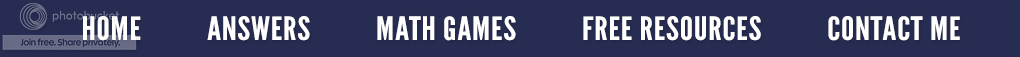### FREE Resources!

The following items in my
Teachers Pay Teachers
store are always FREE.Alphabet + Number Tiles
1) The A, B, C's of Number Tiles - FREE Version -The three letters of this 10 page free resource are solved just like magic squares are. Number tiles are arranged in such a manner that the sum of the tiles that form each straight line of the letter equal the same sum. The designated sum for the letter is written on each page as well as the number tiles to be used. Most of the three letter puzzles have more than one answer; so, students are challenged to find a variety of solutions.  Answer recording sheets are provided for the student as well as possible solutions for the teacher. A Number Tile Keeper in addition to a blackline of the number tiles is located at the end of this 10 page resource.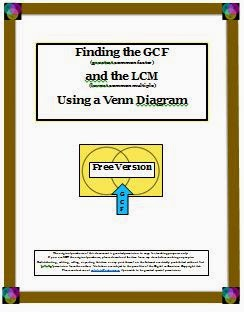2) Algebraic Terms/Fractions - Finding the Greatest Common Factor and Least Common Mulitple - FREE Version

This FREE resource begins with the definitions for the words factor, greatest common factor and least common multiple. After finding the GCF, how to find the least common multiple is explained and demonstrated by using the same Venn Diagram. A step-by-step example is given, followed by two student practice problems. Three blank two circle Venn Diagrams are included.

Also incorporated into this resource is how to use the two circle graphic organizer to factor out the Greatest Common Factor for polynomials or algebraic terms. A detailed example is provided to show how this same process can be used in algebra.

What if there are three numbers? A three circle Venn Diagram would be used. Two blank, three circle Venn Diagrams are added to the resource so that the students can practice. An answer key is included.
common multiple. After finding the GCF, how to find the least common multiple is explained and demonstrated by using the same Venn Diagram. A step-by-step example is given, followed by two student practice problems. Three blank two circle Venn Diagrams are included.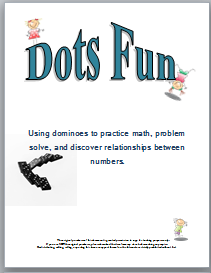Uses Dominoes
3) Dots Fun - FREE Primary Grades Resource (using dominoes) -

This ten page primary resource contains three different activities and one game that uses dominoes. The activities range from adding, to problem solving, to Topsy Turvy subtraction problems. Students solve the Dots Fun Activities by arranging dominoes. You may use a commercial set of 28 or copy the domino blackline included on the last page. These activities are suitable for a math center, for differentiated instruction, as well as for introducing or reviewing a math concept. They correlate well with the math series, Everyday Math.For Grades 4-7
4) Dots Fun for Everyone - Three Activities and One Game that Uses Dominoes - This ten page resource for grades 3-6 contains three different activities and one game . The activities include sorting dominoes, four digitplace value, and using division facts. The game involves finding sums that equal 12. The activities and games vary in difficulty; so differentiated instruction is easy. This resource is also excellent for math center activities and correlates well with the math series, Everyday Math.Geometry + Number Tiles
5)
Learning About Plane Geometry While Using Number Tiles - All of the pages of this 10 page FREE handout are solved like magic squares. Number tiles are positioned so that the total of the tiles on each line of the geometric shape must add up to be the same sum. Most of the geometric puzzles have more than one  answer; so, students are challenged to find a variety of solutions.  Before each set of activities, the geometry vocabulary used for that group is listed. The definitions include diagrams and/or illustrations so that the students can learn and understand new math words without difficulty or cumbersome words.

Ten number tiles are required for each of these four puzzles. The students use each number tile only once to solve each activity. However, some of the activities will not require the use of all ten tiles; so, reading and following the directions before beginning each geometric puzzle is imperative. An answer recording sheet is provided for the student as well as possible solutions for the teacher. A number tile blackline is a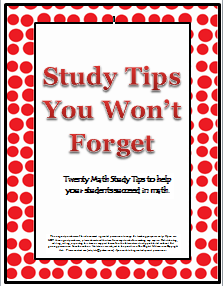6) Math Study Tips You Won't Forget - Math courses are not like other courses. To pass most other subjects, a student must read, understand, and recall the subject matter. However, to pass math, an extra step is required: a student must use the information they have learned to solve math problems correctly. Special math study skills are needed to help the student learn more and to get better grades. This resource lists 20 math study skills intended to help students succeed in math. Use the resource as a journal topic, as a student guide, or copy it and make it into a poster.

7) Numbers for Show Me Boards - This sheet of numbers was created to be used with the Show Me Boards described in the September 7, 2011 blog posting entitled: There’s A Place for Us.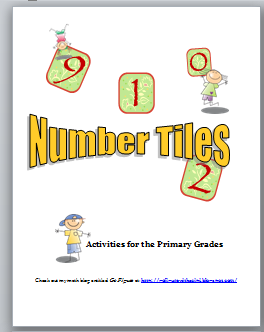8) Number Tiles - Problem Solving Activities for the Primary Grades - This free resource is a ten page booklet containing six different math problem solving activities for the primary grades. The activities extend from simple counting, to greater than or less than to solving addition and subtraction problems. Each activity is on a single page, and varies in difficulty which is appropriate for any diverse classroom. These activities work well for the visual and/or kinesthetic learner.

9) Number Tiles - Problem Solving Math Activities for Grades 5-8 - This seven page resource contains four different math problem solving activities that extend from addition and multiplication, to using the divisibility rules. Since the students do not write in the book, the pages can be copied and laminated so that they can be used from year to year. Students solve the Number Tile Math Activities by arranging ten number tiles, numbered 0-9. Each problem is given on a single page, and since the students have the freedom to move the tiles around, they are more engaged and more willing to try multiple methods to find the solution.Great for Earth Day
10) Trash to Treasure - This FREE Trash to Treasure handout is an eight page booklet that features clever ideas, fun and engaging mini-lessons in addition to cute and easy to construct crafts made from recycled or common, everyday items. In this resource, discover how to take old, discarded materials and make them into new, useful, inexpensive products or tools for your classroom.

Learn many out-of-the-ordinary ways to use milk lids for math. Did you know two plastic beverage lids can be made into card holders for kindergartners or for those whose hands are disabled? Discover how to use butter tubs to create a fun indoor recess game that practices math skills. How about making fun math games out of egg cartons? Because these numerous activities vary in difficulty and complexity, they are appropriate for any PreK-3rd classroom, and the visual and/or kinesthetic learners will love them.Investigation Checklist
11) Student Self-Checklist follows the six steps of the scientific method:

a) Exploration
b) Plan
c) Question
d) Prediction
e) Data Collection and Display
f ) Conclusion

Students simply check the appropriate boxes in each category as they work through an investigation. Since the checklist is generic, it can be used with any science investigation for grades 2-4. Students should utilize it during the entire investigation to help self-check and guide them as they complete a science investigation.12) This free resource lists several different mnemonic devices that will assist students in remembering and recalling larger pieces of information for tests. Included are acronyms, initialism, acrostics, rhyme, rhythm and song and association in addition to visualization using the loci and peg systems. Definitions for each mnemonic method are provided under each category as well as specific mnemonic examples for math, science and language arts.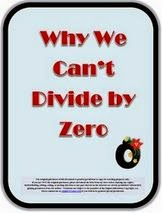13) One day, a student of mine, a mathphobic to be sure, asked, “Why can’t I divide by zero?” Promptly I gave him the answer that any good mathematician would. “You can’t divide by zero because 0 is undefined. It just cannot be done.” Well, that went over like a lead balloon; so, I knew it was time to sit and down and write out a plausible but understandable explanation that my students could understand.

That is what this two page free resource is - a sequential step-by-step explanation of why you cannot divide by zero. It starts off out by explaining what division is and how it relates to multiplication. It concludes with a simple visual to help the students remember the difference between 0 being in the numerator and 0 being in the denominator.